# Characteristic Impedance

Click here to go to our page on light, phase and group velocities

Click here to go to our basic concepts of microwave engineering page

This page and the general topic of Z0 is divided into the following subtopics:

What is characteristic impedance

Lumped element transmission line model

Relationship of L' and C' to Z0 and VP

Telegrapher's equations

Intrinsic impedance

Impedance of of free space

Even and odd mode impedances (separate page)

Measuring Z0 (separate page)

Waveguide wave impedance (new for January 2016!)

### What is charactersitic impedance?

Note to readers.. if you want more discussion on this topic we suggest you pick up a copy of the latest revision of Pozar's Microwave Engineering, look for it on our book page.

Any media that can support a electromagnetic wave has a characteristic impedance associated with it. Although characteristic impedance units are in Ohms, it is not a "real" impedance you can measure using direct current equipment such as a DC Ohmmeter. And although transmission lines have real loss at microwave frequencies, this isn't what we're talking about either.

Let's add one caveat to the above paragraph.... waveguide must be treated differently from this whole discussion of characteristic impedance, go here to learn about waveguide "wave impedance". Technically, waveguide isn't really a transmission line, even though we often treat it as one!

The best way to think about characteristic impedance it envision an infinitely long transmission line, which means that there will be no reflections from the load. Placing an alternating current voltage Vin(t) will result in a current Iin(t). The impedance of the transmission line is then: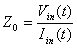Sounds simple enough, but unless you are dealing with "free space", there is no transmission line that is infinitely long. But that equation is starting to look like a version of Ohm's law, where R=V/I.

### Transmission line lumped element equivalent circuit

The transmission line model now has its own page.

Now let's look at the general equivalent circuit of an infinitesimally small piece of a transmission line. All circuits elements are normalized to length in transmission line models; in the metric system the units are Ohms/meter, Farads/meter, mhos/meter and Henries per meter, we will use the "prime" notation when we are discussing quantities that are normalized per unit length.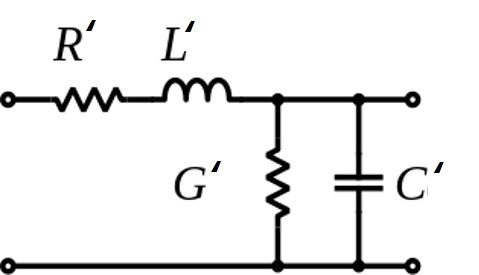The T-line model is repeated infinite times along the length of a real transmission line. Hmm, this is starting to sound like calculus, which we have pledged to avoid on Microwaves101. And we will, so we'll stop with this one section. For microwave engineers, the general expression that defines characteristic impedance is: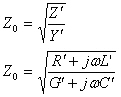Here R', G', L' and C' are normalized to length, the same as in the model. Note that in its general form, characteristic impedance can be a complex number. Also note that it only becomes complex if either R' or G' are non-zero, which will give you a headache if you think about it too long. In practice we try to achieve nearly lossless transmission lines. For a low-loss transmission line, the following relationships will occur: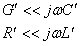Then for all practical purposes we can ignore the contributions of R' and G' from the equation and end up with a nice scalar quantity for characteristic impedance. For lossless transmission lines the transmission line model reduces to this: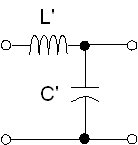and the more familiar equation for characteristic impedance is simply: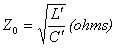What are L' and C' to the lay person? L' is the tendency of a transmission line to oppose a change in current, while C' is the tendency of a transmission line to oppose a change in voltage. Characteristic impedance is a measure of the balance between the two. How do we calculate L' and C'? that depends on what the transmission line is. For example our page on coax give the coax equations.

### Relationship of L' and C' to Z0 and VP

There are many situations where you need to know inductance per unit length and capacitance per unit length of a transmission line. Both can be calculated from the characteristic impedance and the propagation velocity of the wave in a transmission line. The key to solving these equations is that the propagation velocity of a transmission line is a very simple function of its capacitance and inductance per unit length: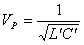Note than when you plug in inductance in Henries/meter and capacitance in Farads/meter, you are talking about one speedy wave, limited to be less than or equal to the velocity of light which is about 3x10E8 meters/second. From this equation and that for Z0 (above) you can arrive at the following for L' and C':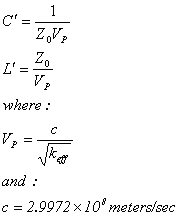Now the truth comes out... for a TEM transmission line such as stripline or coax, and for a given dielectric material (which would correspond to Keff in the above equations), the inductance and capacitance per unit length don't change when you scale the geometry up and down. So all semirigid, 50 ohm PTFE-filled cables (and PTFE-filled stripline!) will have 94.8 pF/meter (28.9 pF/foot) capacitance and 237 nH/meter (72.2 nH/foot) inductance! Let's state that as a Microwaves101 Rule of Thumb: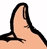For coax and stripline 50 ohm transmission lines that employ PTFE dielectric (or any dielectric material with dielectric constant=2), the inductance per foot of is approximately 70 nH, and the capacitance per foot is about 30 pF.

### Telegrapher's equations

This solution to the wave equation for transmission lines was developed by Oliver Heaviside a long time ago. We'll cover this later. You can look it up in Wikipedia for now!

### Intrinsic impedance

Characteristic impedance does not even need a transmission line, there is a characteristic impedance associated with wave propagation in any uniform medium. In this case we use the Greek letter eta for impedance. The intrinsic impedance is a measure of the ratio of the electric field to the magnetic field.

Intrinsic impedance is calculated the same way as any transmission line. Assuming there are no "real" conductances or resistance in the medium, the equation is reduced to the simpler SQRT(L'/C') form. In this case the inductance per unit length reduces to the permeability of of the medium, and the capacitance per unit length reduces to the permittivity of medium.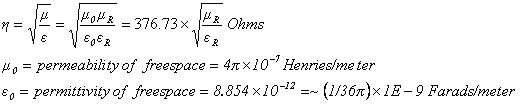Note that the approximation that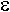0 is related to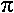. There is no physical reason for this, it just happens that 1/36gives better than 99.9% accuracy!

### Impedance of free space

In space the terms for relative permeability and relative permittivity are each equal to unity, so the intrinsic impedance equation is simplified to the equation for characteristic impedance of free space: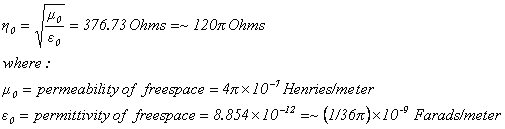Here's where the approximation involving 1/36for permeability is what gives us that 120value for free-space impedance (accurate to 99.9%!). Note that permeability and permittivity of the atmosphere on earth behave very close to free space (if it ain't raining!), so we use 377 ohms for the characteristic impedance of free space in most calculations involving atmospheric propagation.